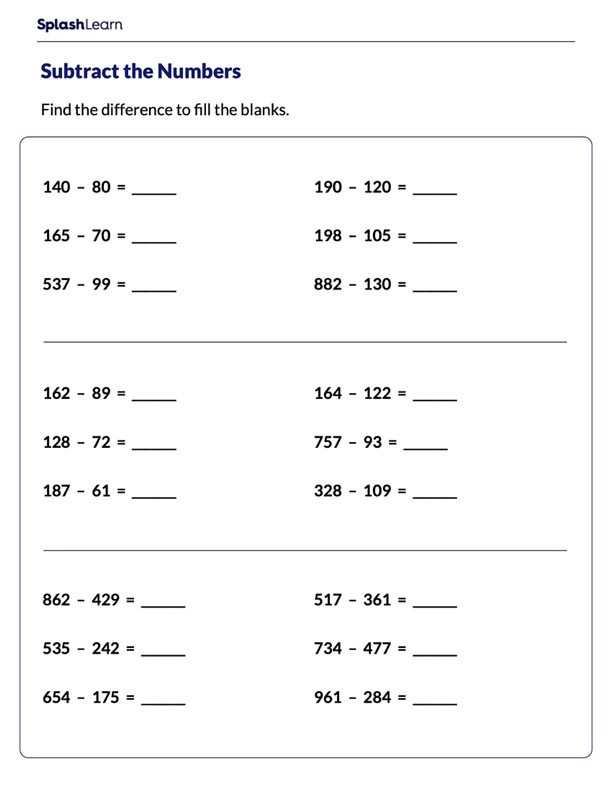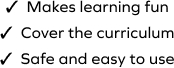# Subtraction Worksheet

SplashLearn - The complete PreK - Grade 5 Math & ELA Learning Program Built for Your Child
Home > Subtraction WorksheetHelp your child learn subtraction strategies in an efficient manner with this challenging worksheet. The worksheet challenges young mathematicians to hone their math skills by solving a set of problems on subtraction to find the missing numbers in math sentences. In this task, learners will practice "make a multiple of 100 to subtract" strategy to solve the problems.